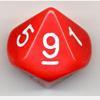# Resources tagged with: Rounding

Filter by: Content type:
Age range:
Challenge level:

There are 9 NRICH Mathematical resources connected to Rounding, you may find related items under The Number System and Place Value.

Broad Topics > The Number System and Place Value > Rounding### Reasoned Roundinglive

##### Age 7 to 14Challenge Level

Four strategy dice games to consolidate pupils' understanding of rounding.### Round the Dice Decimals 1

##### Age 7 to 11Challenge Level

Use two dice to generate two numbers with one decimal place. What happens when you round these numbers to the nearest whole number?### Round the Three Dice

##### Age 7 to 11Challenge Level

What happens when you round these three-digit numbers to the nearest 100?### Round the Two Dice

##### Age 5 to 7Challenge Level

This activity focuses on rounding to the nearest 10.### Round the Dice Decimals 2

##### Age 7 to 11Challenge Level

What happens when you round these numbers to the nearest whole number?### Round the Four Dice

##### Age 7 to 11Challenge Level

This activity involves rounding four-digit numbers to the nearest thousand.### Rough Rectangle

##### Age 11 to 14 ShortChallenge Level

What is the smallest possible area that this rectangle could have?### Archimedes and Numerical Roots

##### Age 14 to 16Challenge Level

The problem is how did Archimedes calculate the lengths of the sides of the polygons which needed him to be able to calculate square roots?### Route to Root

##### Age 16 to 18Challenge Level

A sequence of numbers x1, x2, x3, ... starts with x1 = 2, and, if you know any term xn, you can find the next term xn+1 using the formula: xn+1 = (xn + 3/xn)/2 . Calculate the first six terms of this sequence. What do you notice? Calculate a few more terms and find the squares of the terms. Can you prove that the special property you notice about this sequence will apply to all the later terms of the sequence? Write down a formula to give an approximation to the cube root of a number and test it for the cube root of 3 and the cube root of 8. How many terms of the sequence do you have to take before you get the cube root of 8 correct to as many decimal places as your calculator will give? What happens when you try this method for fourth roots or fifth roots etc.?